Custom Writing Service

Price from

## £84.99

- Rates starting at £0.08 per word

- Free revisions

Delivered on-time or your money back

##### Rated 4.8 / 5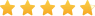### Popular Services

Dissertation

Essay

Assignment

Dissertation Proposal

Editing & Improvement

# Statistics Project (Scenario Based)

Disclaimer: This is not a sample of our professional work. The paper has been produced by a student. You can view samples of our work here. Opinions, suggestions, recommendations and results in this piece are those of the author and should not be taken as our company views.

Type of Academic Paper – Essay

Word Count – 2000 words

## Introduction

In this document, the data analysis has been done in order to assess advanced statistical analysis. The first part consists of the Analysis of variance that is also known as ANOVA where the attractiveness ratings are considered as the discrete predictor or independent variable. The second part consists of a regression model where the two independent variables are attractiveness as a continuous predictor and attractiveness ratings as a discrete predictor in order to get attractiveness level. The third part consists of the linear mixed model where the same continuous and discrete predictors are used in order to form mixed model with fixed effect and mixed model with random effect. A random intercept has also been predicted in the analysis for both raters (female participants) and rated (male confederates). The document also contains a Bayesian analysis and power analysis. In the end, the conclusion of the whole discussion is present.

## Standard Analysis of Variance with Rating Condition as a Discrete Predictor

The following analysis is conducted with regard to rating condition as the discrete predictor. The situation was that a group of researchers was trying to determine about how likely people agree to date unattractive people out of pity. The female participants in this study were the raters whereas 20 male were used as rated. The rating was done on the basis of how likely are females are going to date, unattractive people.  Analysis of variance or more commonly called as ANOVA was used for assessing the results of this study.

On the basis of the application of ANOVA, it was found that rating condition used as a discrete predictor was found to be significant. The table below shows the output of ANOVA obtained from Excel. The first chart of summary shows variance and the next table below shows ANOVA.  The value of F-statistic in the given table is greater than the critical value of F-statistic which means that the hypothesis of the study that females date unattractive people out of pity was rejected the null hypothesis. The P-value shows is 0 which means that the results are highly significant and the females mostly date males out of pity if they are unattractive. Furthermore, the variance shows that the values deviate from the mean.  The P-value also shows that the ANOVA shows significant results of the data. In addition to this, it was also found from the below table that the research conducted by the researchers was validated because the results are significant. However, if the results were not significant and the value of P had been more than 0.05 so, the research was declared to be not successful.

The table below also shows that ANOVA is a statistical technique which is helpful in understanding the variance between groups of means.  The ANOVA also shows the variance within the groups and between the groups that are taken under consideration for analysis. There are two type of ANOVA but for the following study, the researcher had conducted one-way ANOVA only so that results can be obtained easily.  The rating condition was taken as the discrete predictor because the researcher had used attractiveness as one variable and the other was rating done to the number of dates females go if they males are unattractive. Moreover, the attractiveness of the male gender was not considered as the dependent variable because it depends on the choice of the females to select males.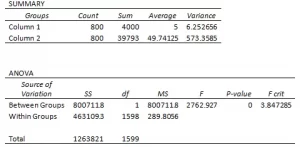## Standard Multiple Regression Model with Rating Condition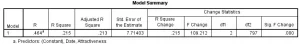Regression analysis is defined as the statistical method used for determining the relationship between independent and dependent variables. The purpose of using regression analysis is to validate whether any association exist between two variables. SPSS and Microsoft Excel are used to find out the relationship through regression analysis.

The second part of the situation was to conduct standard multiple regression model with rating condition. SPSS was used to obtain results for multiple regressions.  Three tables were obtained: model summary, coefficient and ANOVA. The first table above of Model summary shows that the R value was 46.4% and R-square was 21.5% however, the significant F-change was 0.000. The values of R and R square show weak results which mean that in comparison with the analysis of variance conducted in the earlier section, the R value and r square are weak which do not validate the results. Furthermore, the results in the above table also show that rating condition cannot be validated accurately as the model is not very fit for analysis.The second table of regression analysis is ANOVA. It shows the variance between group of means. The most important value taken under consideration in the above table is significance value. The table of ANOVA shows that the value of sig or the P-value was 0.000 that is less than the alpha value of 0.05 hence it validates significant results.  Attractiveness was taken in this study as the independent variable and rater was used as the dependent variable. The results evidently show that the F-statistic is high and the sig-value is also 0.000 so it means that rater and attractiveness has relationship.

The third table of regression analysis was coefficients. It shows the significant value of the variables taken for analysis. The significance value was 0.000 which show that the results are significant and that there is strong association. Rater and attractiveness has relationship because the attractiveness of female increases if the male are not attractive and they go on date for pity. The table of coefficients of determination is very important for regression analysis as it helps in the analysis and understanding of the increase in the dependent variable with one unit increase in the independent variable.The third table of regression analysis was coefficients. It shows the significant value of the variables taken for analysis. The significance value was 0.000 which show that the results are significant and that there is strong association. Rater and attractiveness has relationship because the attractiveness of female increases if the male are not attractive and they go on date for pity. The table of coefficients of determination is very important for regression analysis as it helps in the analysis and understanding of the increase in the dependent variable with one unit increase in the independent variable.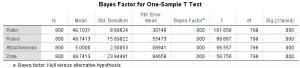The second test applied in this study was Bayes factor for One-Sample t -test. The table above shows that sample size was 800, the standard deviation and mean along with significant value. It also makes the assumption that the data or the observations made are all normally distributed.  The significant value is 0.000 which is less than 0.005 meaning that the observations made are all normally distributed and are significant.  The Bayes factor in the table above is also 0.000 for rater, rated, attractiveness and the date. Hence, it means that how likely people are to agree to date unattractive people out of pity is positive and significant.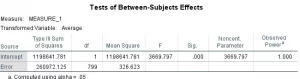The tests of analysis used for this study was tests between-subject effects to understand the power value. The table shows that overall observed power was 1.000 whereas the significant value, in this case, was also 0.000. It is basically the power of the applied tests which is derived on the basis of the size of the total data and observations. The main purpose of using these tests is not to focus on the power but focus on the size of the data used.

## Hire an Expert Writer

Orders completed by our expert writers are

• Formally drafted in an academic style
• Free Amendments and 100% Plagiarism Free – or your money back!
• 100% Confidential and Timely Delivery!
• Free anti-plagiarism report
• Appreciated by thousands of clients. Check client reviews## Linear Mixed Model

Mixed model is helpful in analyzing a variety and range of correlation patterns. There are both effects incorporated in the mixed model that are random and fixed effects. A linear mixed model is the one that is one dimensional and has degree equal to one. It is nothing but an extension to the general linear models. If the values of the variables of the interest are present in the data set then those are called fixed effects whereas; if the values are random in the given dataset then those are called random effects.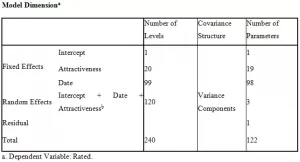The table mentioned above shows a mixed model with random and fixed effects and is also known as model dimension. The dependent variable that is taken is rated (male confederates) who were rated by the female participants on the basis of their level of attractiveness. The total term in the model shows the overall complexity of the model especially in the column of number of parameters. The column of number of level shows that how much lines are dedicated to explanatory variable that can easily be seen above. The residual number of parameter shows that how much data was out of reach for estimated parameters and was completely blank. The intercept value shows the number of random effects. Here in model we have one random effect that can be seen in the table.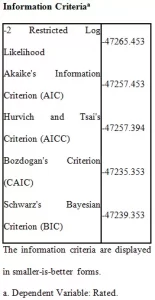The above table shows the information criteria of the linear mixed model. The focus is more on the Schwarz’s Bayesian Criterion (BIC) that is quite small and negative means that the model is good. The small value of AIC or BIC indicates that the model is more adequate. These information criterions are generally taken as universal parameter of adequacy of the model. This shows that the model based on the dependent variables as the male confederates (rated variable) is highly significant. It depicts that attractiveness does have impact on the female participants to date that person.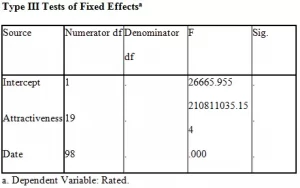The table shows a test for fixed effects model and it is similar to ANOVA table. The f value indicates that the model is significant where the random variable is taken as rated male confederates on an average.

On the basis of rater that are the female participants who rated the male on their level of attractiveness and likelihood to date them, following results were obtained:Here, with respect to rater variable as dependent, the results are quite similar as rated variable. The complexity of the model is quite high. The residual number of parameter is also one which means that there was only one estimated parameter that was beyond the range during the analysis. However, the taken dependent variable is the female participants who rated male colleagues.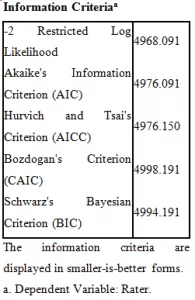The Schwarz’s Bayesian Criterion (BIC) is quite high which means that with respect to rater dependent variable, the model does not seem to be effective. The lower the value of Bayesian Information Criterion (BIC), the better the model is and similar is the case with Akaike’s Information Criteria (AIC). The value of AIC is also not appealing so, it is evident that this model is not a good fit model for the attractiveness of male confederates with respect to the data of ratings provided by the female participants on attractiveness. So, this model does not seem to be adequate.## Conclusion

In the analysis aggregately, various tests are being run and various aspects are tested. It can be concluded that the linear regression model seem to be more accurate. On the basis of R-squared, the linear regression analysis with respect to rated (the male confederates) seems to be more accurate which concludes that the model is best fit. On the basis of mixed model, the model does not appear to be significant enough but the model with that has dependent variable as rated (male confederates) is significant on the basis of lowest BIC value. The two models validated that the results with respect to rated male confederates as dependent variable are significant and the attractiveness of male confederates matter for the female participants to date their male colleagues or allies.

### DMCA / Removal Request

If you are the original writer of this essay and no longer wish to have the essay published on the www.ResearchProspect.com then please: